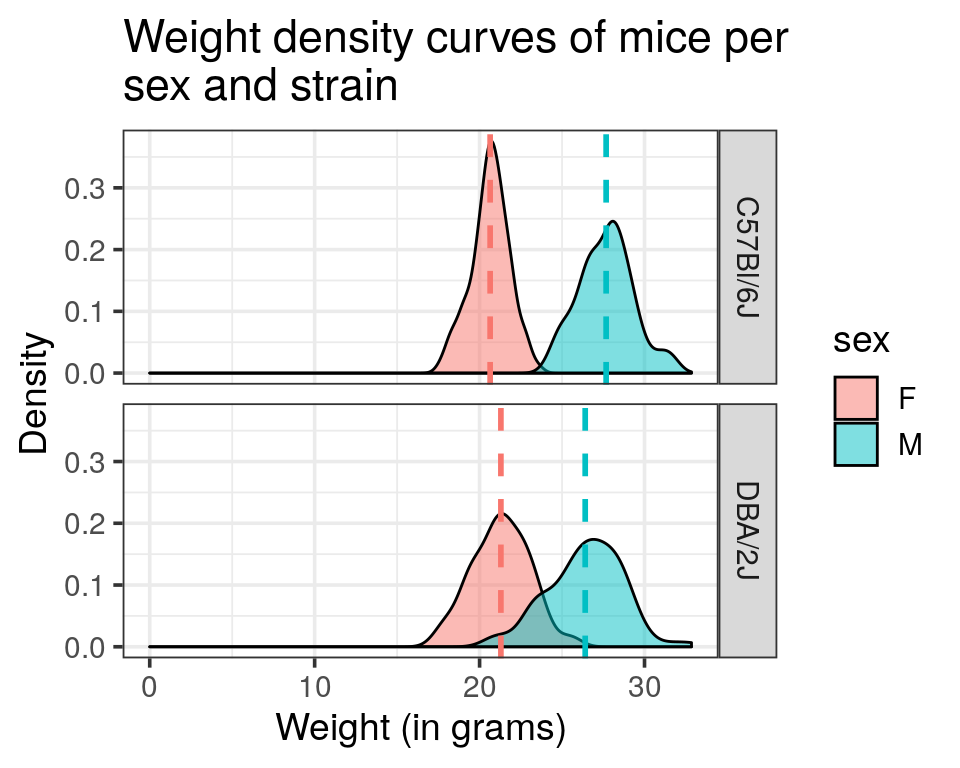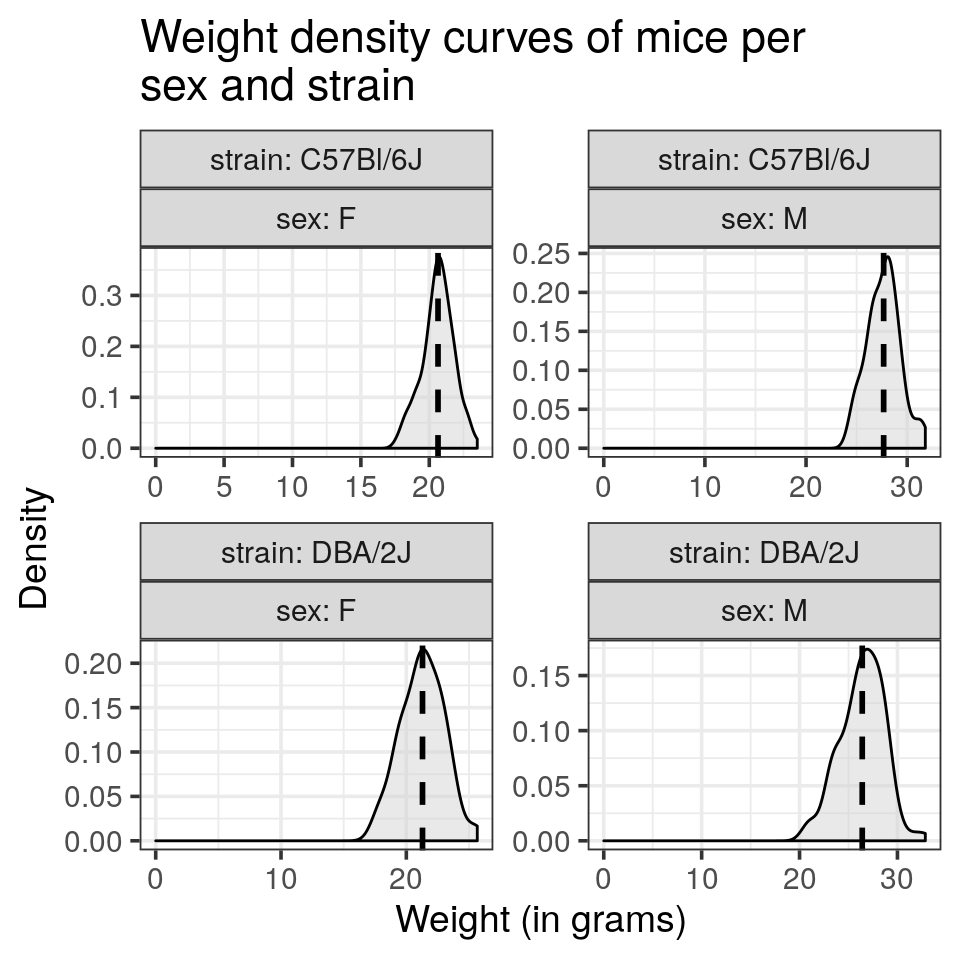This tutorial is inspired and adapted from the sthda practical guide published under the creative commons license.

# Mouse weights

Download the mousew dataset from here and load it in R. This dataset contains the weight (in grams) of two strains of mice for both genders.## Histogram and density curve representations

1. Draw a histogram of the weight distribution and adjust the range for every bar to 0.5 g.

2. Attribute a color to the different genders histograms
• Have a look at the overlapping region. Could you find a way to avoid the stacking of bars?

#### Tip

Have a look at the position argument.
1. Draw a density curve of the weight for both genders.

2. Overlay the histogram representation to the previous plot.

3. The data frame contains the weight of 2 mouse strains: Split the plot into two separate ones for each strain and draw one above the other (in rows).
4. Add a vertical dashed line representing the mean value of each group
5. ggplot2 automatically adjusts the range of the axis. Try to override this behaviour and let the x axis start at 0
6. Draw each density curve of sex and mouse strain combination in a single panel.
7. Try to reproduce the following plot:
• Would you recommend these settings to display the weight distributions?

#### Tip

remember that each geom_* can takes its own data argument that overwrite the one inherited from the ggplot() call. Might be worth summarising by the mean the weight and a 4 rows tibble. Then pass it to geom_vline(). Of note, aesthetics are also not inherited when a new data is specified.

#### Tip

• to get the x axis start at 0, have a look at the expand_limit() function
• labels that recall the variable names (such as strain and sex), see the labeller argument of facet_wrap()1. draw the gender as colour and reproduce the plot displayed as introduction

## Boxplot and bar chart representations

1. Draw a box plot of the weight of rodents for each sex
• use again an additional command to display the y-axis from 0.
2. Draw a bar chart of the weight for each sex, colored by strain

#### Tip

• using geom_col() requires a y aesthetetic to map on the continuous variable
• set the alpha paramater to give some transparency, will help to spot inconsistencies
1. Does it make sense?

2. Draw a bar chart of the summarised weight (with mean) for each sex, colored by strain

#### Tip

mind the position argument for geom_col(), default is stack, alternatives are dodge (side by side) or fill for proportions
1. Add error bars to your bar chart using geom_errorbar() and using the standard deviations (sd).

#### Tip

• you will need to adjust the dodging for error bars. position = "dodge" calls the position_dodge() function. Look at the help of this function, one example is describing how to align narrower elements like error bars.
• See the width element of geom_errorbar() to reduce the default which is too large.